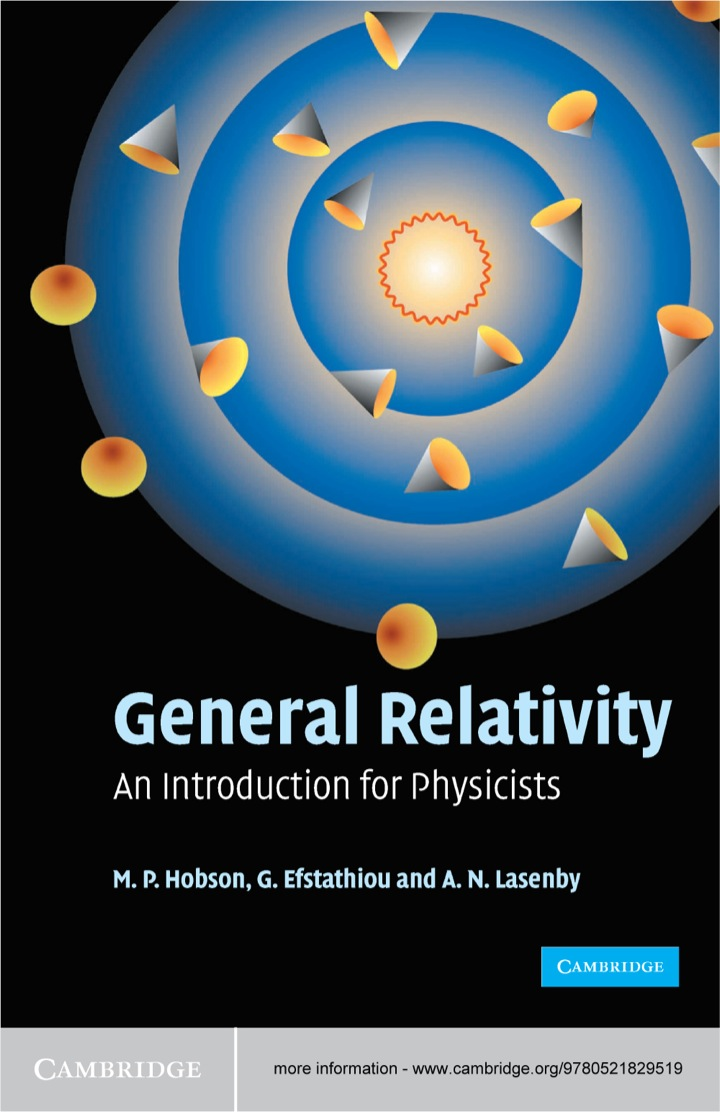# General Relativity

## General Relativity

By M. P. Hobson; G. P. Efstathiou; A. N. Lasenby

### Rent or Buy eTextbook

Expires on Apr 11th, 2022
\$78

Publisher List Price: \$0.00

General Relativity: An Introduction for Physicists provides a clear mathematical introduction to Einstein's theory of general relativity. It presents a wide range of applications of the theory, concentrating on its physical consequences. After reviewing the basic concepts, the authors present a clear and intuitive discussion of the mathematical background, including the necessary tools of tensor calculus and differential geometry. These tools are then used to develop the topic of special relativity and to discuss electromagnetism in Minkowski spacetime. Gravitation as spacetime curvature is then introduced and the field equations of general relativity derived. After applying the theory to a wide range of physical situations, the book concludes with a brief discussion of classical field theory and the derivation of general relativity from a variational principle. Written for advanced undergraduate and graduate students, this approachable textbook contains over 300 exercises to illuminate and extend the discussion in the text.

Subject: Physical & Earth Sciences -> Astronomy -> Stars & GalaxiesAn Introduction for Physicists
1st edition
Publisher: Cambridge University Press 2/2/06
Imprint: Cambridge University Press
Language: English

ISBN 10: 1139637096
ISBN 13: 9781139637091
Print ISBN: 9780521829519

Live Chats